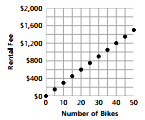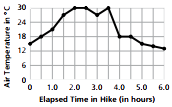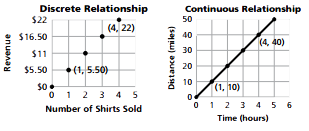Display Accessibility Tools

# 6.6 Variables and Patterns - Concepts and Explanations

## Variables

A variable is a quantity that can change. Letters are often used as symbols to represent variables in rules that describe patterns.

### Example

The number of students, n, who go on a trip is related to the price of the trip, p, for each student.

## Patterns

A pattern is change that occurs in a predictable way. Students work on problems that require them to predict the pattern of change in values of one variable, as it relates to changes in values of another variable.

### ExampleAs the number of bikes increases by 1, the rental fee increases by \$30.

## Tables

A table is a list of values for two or more variables that shows the relationship between them. A table may show a pattern of change between two variables that can be used to predict values for other entries in the table.

The table shows how a change in one variable affects the change in the other variable.

### Example

As the number of campsites, x, changes by one unit, the total campground fee, y, changes by 12.5 units.  The table can be continued by adding 1 to the previous entry in the x row and adding \$12.50 dollars to the previous entry in the y row.

 Number of campsites Total campground fee 1 2 3 4 5 6 7 8 \$12.50 \$25.00 \$37.50 \$50.00 \$62.50 \$75.00 \$87.50 \$100.00

## Coordinate Graphs

A coordinate graph shows a representation of pairs of related numerical values. It relates the independent variable (shown on the x-axis) and the dependent variable (shown on the y-axis).

Graphs are another way to view the patterns of change between the variables.

# Example## Discrete vs. Continuous Data

From a statistical perspective, there are two basic types of quantitative variables—those with only a countable set of values (discrete data) and those with real-number values (continuous data). Tables can only represent discrete collections of (x, y) values. Graphs can represent both but often suggest continuous variables.

### ExampleFor the graph on the left, the number of shirts sold and revenue is a discrete relationship. Connecting two points does not make sense. It would imply that part of a shirt could be sold.

For the graph on the right, situations such as the distance/time/rate relation are continuous.  If a bicyclist peddles at a rate of 10 miles per hour, it is reasonable to connect the points, because you can go a distance in part of an hour.

## Rules and Equations

Rules are a summary of a predictable relationship that tells how to find the values of a variable. A rule may be given in words or as an equation. Equations (or formulas) are rules containing variables

The advantage of a symbolic rule is that it is brief and represents a complete picture of the pattern, while tables and graphs can represent only parts of the relationships.

### Example

These rules relate time, rate, and distance: distance is equal to rate times time d = rt

Rule (in words): Total profit equals profit per T-shirt times the number of shirts sold.

Rule (written as an Equation): y = 10x

A formula or equation for finding the area of a circle: A = Πr2